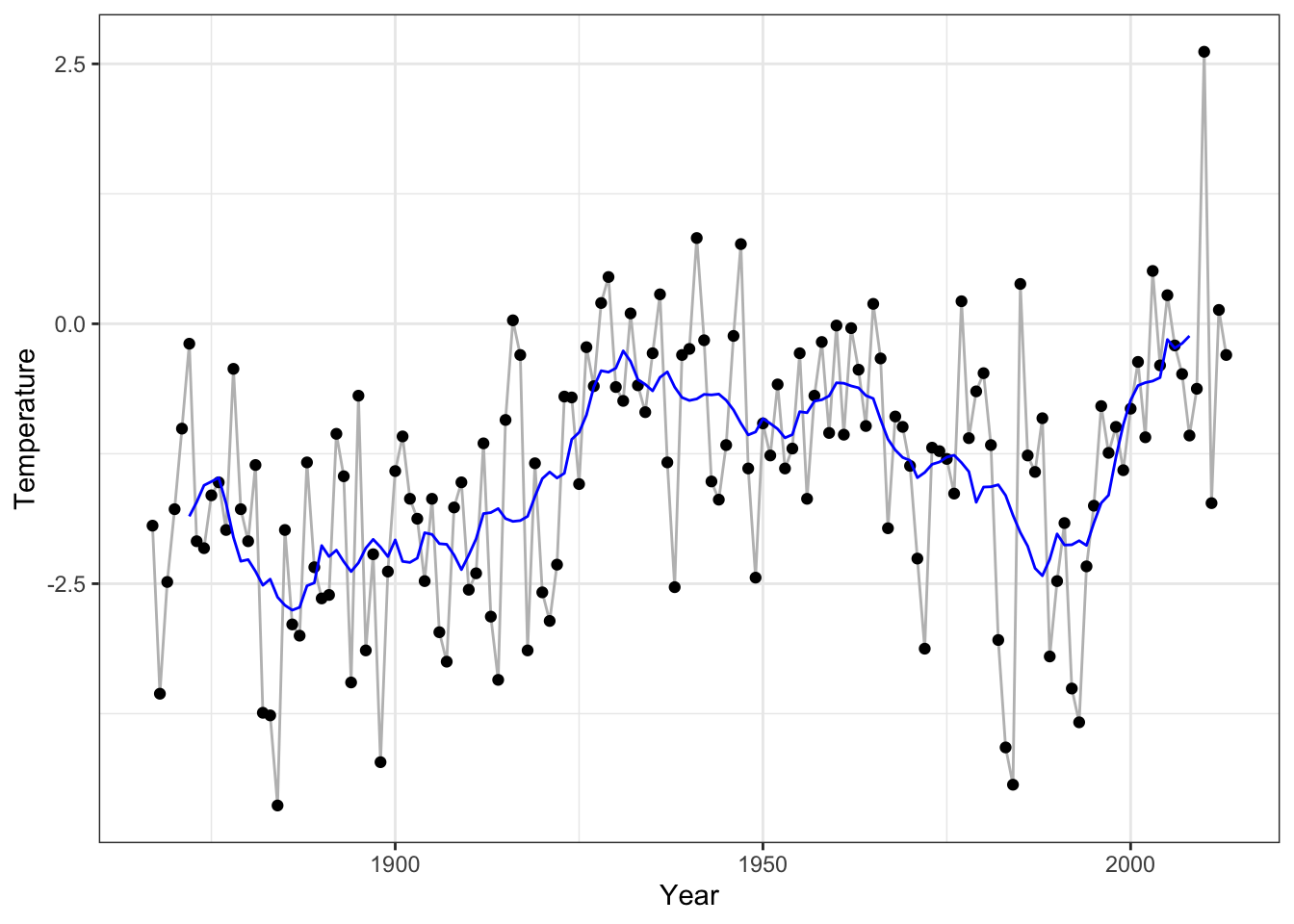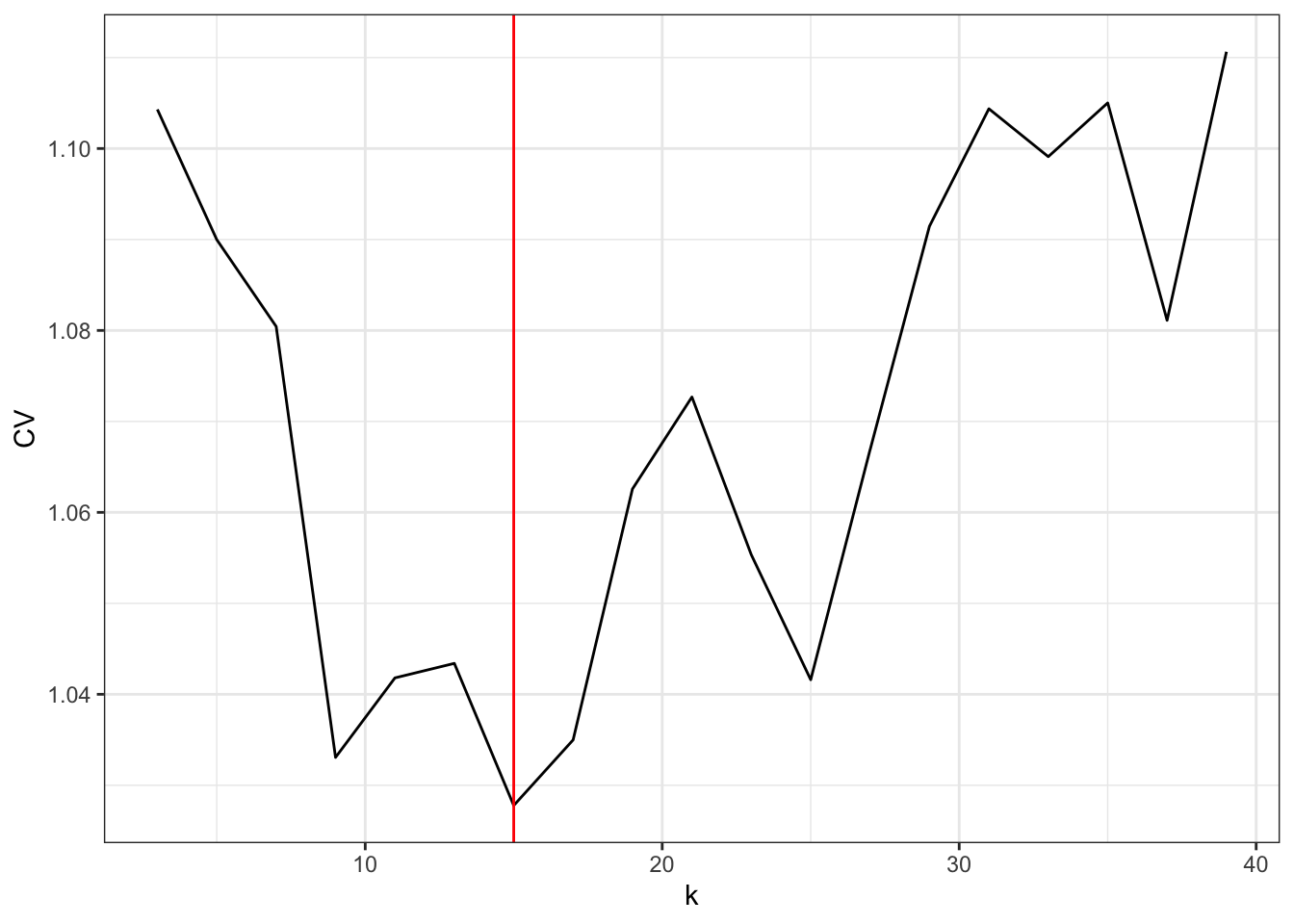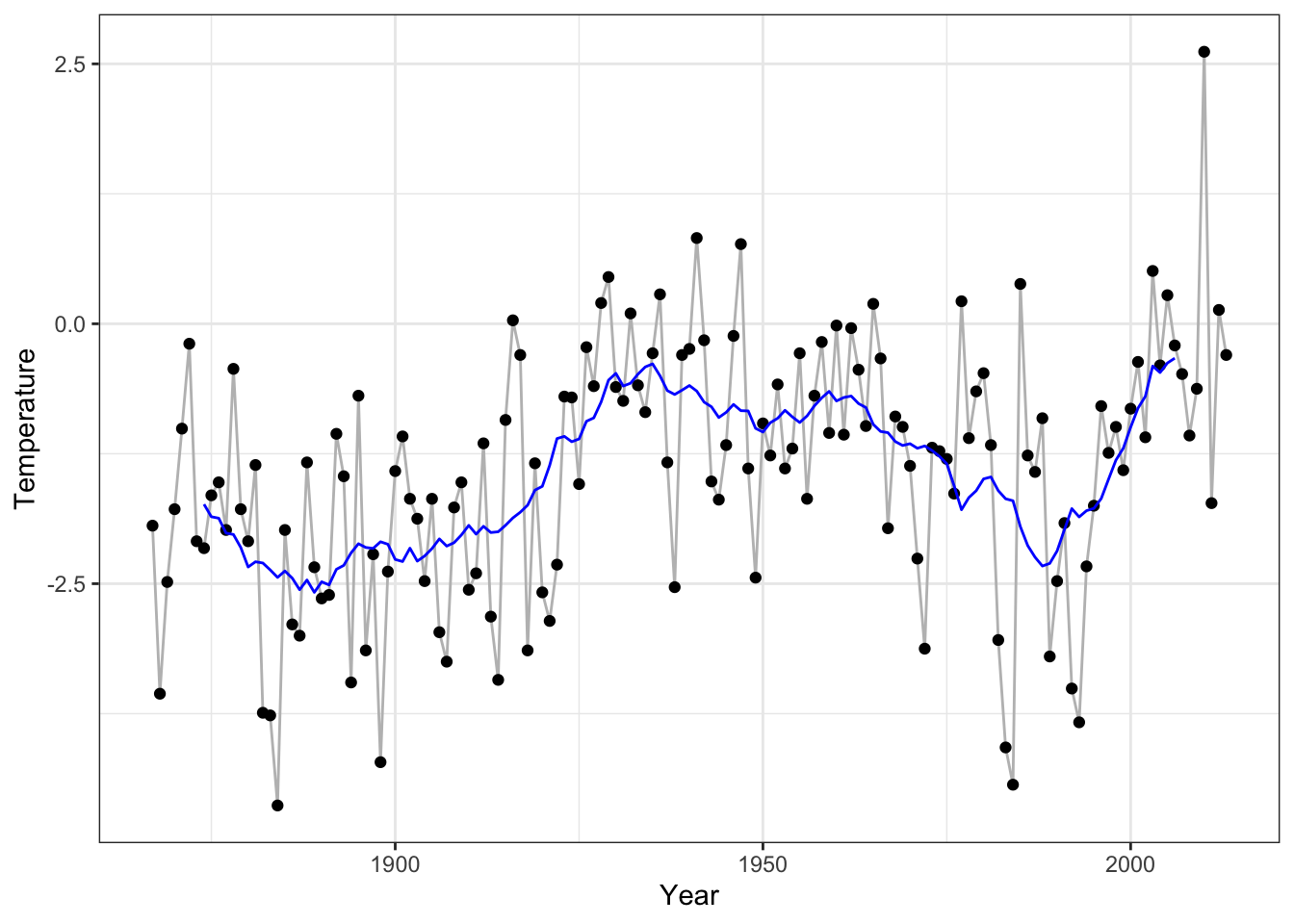## 3.1 Nearest neighbor smoothers

One of the most basic ideas on smoothing bivariate data is to use a running mean or moving average. This is particularly sensible when the $$x$$-values are equidistant, e.g. when the observations constitute a time series such as the Nuuk temperature data. The running mean is an example of the more general nearest neighbor smoothers.

Mathematically, the $$k$$ nearest neighbor smoother in $$x_i$$ is defined as $\hat{f}_i = \frac{1}{k} \sum_{j \in N_i} y_j$ where $$N_i$$ is the set of indices for the $$k$$ nearest neighbors of $$x_i$$. This simple idea is actually very general and powerful. It works as long as the $$x$$-values lie in a metric space, and by letting $$k$$ grow with $$n$$ it is possible to construct consistent nonparametric estimators of regression functions, $$f(x) = E(Y \mid X = x)$$, under minimal assumptions. The practical problem is that $$k$$ must grow slowly in high dimensions, and the estimator is not a panacea.

In this chapter we focus exclusively on $$x$$ being real valued with the ordinary metric used to define the nearest neighbors. The total ordering of the real line adds a couple of extra possibilities to the definition of $$N_i$$. When $$k$$ is odd, the symmetric nearest neighbor smoother takes $$N_i$$ to consist of $$x_i$$ together with the $$(k-1)/2$$ smaller $$x_j$$-s closest to $$x_i$$ and the $$(k-1)/2$$ larger $$x_j$$-s closest to $$x_i$$. It is also possible to choose a one-sided smoother with $$N_i$$ corresponding to the $$k$$ smaller $$x_j$$-s closest to $$x_i$$, in which case the smoother would be known as a causal filter.

The symmetric definition of neighbors makes it very easy to handle the neighbors computationally; we don’t need to compute and keep track of the $$n^2$$ pairwise distances between the $$x_i$$-s, we only need to sort data according to the $$x$$-values. Once data is sorted, $N_i = \{i - (k - 1) / 2, i - (k - 1) / 2 + 1, \ldots, i - 1 , i, i + 1, \ldots, i + (k - 1) / 2\}$ for $$(k - 1) / 2 \leq i \leq n - (k - 1) / 2$$. The symmetric $$k$$ nearest neighbor smoother is thus a running mean of the $$y$$-values when sorted according to the $$x$$-values. There are a couple of possibilities for handling the boundaries, one being simply to not define a value of $$\hat{f}_i$$ outside of the interval above.

With $$\hat{\mathbf{f}}$$ denoting the vector of smoothed values by a nearest neighbor smoother we can observe that it is always possible to write $$\hat{\mathbf{f}} = \mathbf{S}\mathbf{y}$$ for a matrix $$\mathbf{S}$$. For the symmetric nearest neighbor smoother and with data sorted according to the $$x$$-values, the matrix has the following band diagonal form

$\mathbf{S} = \left( \begin{array}{cccccccccc} \frac{1}{5} & \frac{1}{5} & \frac{1}{5} & \frac{1}{5} & \frac{1}{5} & 0 & 0 & \ldots & 0 & 0 \\ 0 & \frac{1}{5} & \frac{1}{5} & \frac{1}{5} & \frac{1}{5} & \frac{1}{5} & 0 & \ldots & 0 & 0\\ 0 & 0 & \frac{1}{5} & \frac{1}{5} & \frac{1}{5} & \frac{1}{5} & \frac{1}{5} & \ldots & 0 & 0 \\ \vdots & \vdots & \vdots & \vdots & \vdots & \vdots & \vdots & \ldots & \vdots & \vdots \\ 0 & 0 & 0 & 0 & 0 & 0 & 0 & \ldots & \frac{1}{5} & \frac{1}{5} \\ \end{array} \right)$

here given for $$k = 5$$ and with dimensions $$(n - 4) \times n$$ due to the undefined boundary values.

### 3.1.1 Linear smoothers

A smoother of the form $$\hat{\mathbf{f}} = \mathbf{S}\mathbf{y}$$ for a smoother matrix $$\mathbf{S}$$, such as the nearest neighbor smoother, is known as a linear smoother. The linear form is often beneficial for theoretical arguments, and many smoothers considered in this chapter will be linear smoothers. For computing $$\mathbf{f}$$ there may, however, be many alternatives to forming the matrix $$\mathbf{S}$$ and computing the matrix-vector product. Indeed, this is often not the best way to compute the smoothed values.

It is, on the other hand, useful to see how $$\mathbf{S}$$ can be constructed for the symmetric nearest neighbor smoother.

w <- c(rep(1/11, 11), rep(0, 147 - 10))
S <- matrix(w, 147 - 10, 147, byrow = TRUE)
## Warning in matrix(w, 147 - 10, 147, byrow = TRUE): data length  is not a
## sub-multiple or multiple of the number of rows 

The construction above relies on vector recycling of w in the construction of S and the fact that w has length $$147 + 1$$, which will effectively cause w to be translated by one to the right every time it is recycled for a new row. As seen, the code triggers a warning by R, but in this case we get what we want.

SWe can use the matrix to smooth the annual average temperature in Nuuk using a running mean with a window of $$k = 11$$ years. That is, the smoothed temperature at a given year is the average of the temperatures in the period from five years before to five years after. Note that to add the smoothed values to the previous plot we need to pad the values at the boundaries with NAs to get a vector of length 147.

# Check first if data is sorted correctly.
# The test is backwards, but confirms that data isn't unsorted :-)
is.unsorted(Nuuk_year$Year) ##  FALSE f_hat <- c(rep(NA, 5), S %*% Nuuk_year$Temperature, rep(NA, 5))
p_Nuuk + geom_line(aes(y = f_hat), color = "blue")Figure 3.2: Annual average temperature in Nuuk smoothed using the running mean with $$k = 11$$ neighbors.

### 3.1.2 Implementing the running mean

The running mean smoother fulfills the following identity $\hat{f}_{i+1} = \hat{f}_{i} - y_{i - (k-1)/2} / k + y_{i + (k + 1)/2} / k,$ which can be used for a much more efficient implementation than the matrix-vector multiplication. It should be emphasized again that the identity above and the implementation below assume that data is sorted according to $$x$$-values.

# The vector 'y' must be sorted according to the x-values
run_mean <- function(y, k) {
n <- length(y)
m <- floor((k - 1) / 2)
k <- 2 * m + 1           # Ensures k to be odd and m = (k - 1) / 2
y <- y / k
s <- rep(NA, n)
s[m + 1] <- sum(y[1:k])
for(i in (m + 1):(n - m - 1))
s[i + 1] <- s[i] - y[i - m] + y[i + 1 + m]
s
}
p_Nuuk + geom_line(aes(y = run_mean(Nuuk_year$Temperature, 11)), color = "blue")Figure 3.3: Annual average temperature in Nuuk smoothed using the running mean with $$k = 11$$ neighbors. This time using a different implementation than in Figure 3.2. The R function filter() (from the stats package) can be used to compute running means and general moving averages using any weight vector. We compare our two implementations to filter(). f_hat_filter <- stats::filter(Nuuk_year$Temperature, rep(1/11, 11))
range(f_hat_filter - f_hat, na.rm = TRUE)
##  -4.440892e-16  4.440892e-16
range(f_hat_filter - run_mean(Nuuk_year$Temperature, 11), na.rm = TRUE) ##  -1.332268e-15 4.440892e-16 Note that filter() uses the same boundary convention as used in run_mean(). A benchmark comparison between matrix-vector multiplication, run_mean() and filter() gives the following table with median run time in microseconds. ## expr median ## 1 S1 %*% y[1:512] 408.7950 ## 2 S2 %*% y[1:1024] 1700.9515 ## 3 S3 %*% y[1:2048] 6847.7495 ## 4 S4 %*% y[1:4096] 27651.4370 ## 5 run_mean(y[1:512], k = 11) 81.3415 ## 6 run_mean(y[1:1024], k = 11) 149.2005 ## 7 run_mean(y[1:2048], k = 11) 296.2385 ## 8 run_mean(y[1:4096], k = 11) 621.4225 ## 9 stats::filter(y[1:512], rep(1/11, 11)) 120.6280 ## 10 stats::filter(y[1:1024], rep(1/11, 11)) 135.5900 ## 11 stats::filter(y[1:2048], rep(1/11, 11)) 200.1870 ## 12 stats::filter(y[1:4096], rep(1/11, 11)) 325.7340 The matrix-vector computation is clearly much slower than the two alternatives, and the time to construct the $$\mathbf{S}$$-matrix has not even been included in the benchmark above. There is also a difference in how the matrix-vector multiplication scales with the size of data compared to the alternatives. Whenever the data size doubles the run time approximately doubles for both filter() and run_mean(), while it quadruples for the matrix-vector multiplication. This shows the difference between an algorithm that scales like $$O(n)$$ and an algorithm that scales like $$O(n^2)$$ as the matrix-vector product does. Despite that filter() is implementing a more general algorithm than run_mean(), it is still faster, which reflects that it is implemented in C and compiled. ### 3.1.3 Choose $$k$$ by cross-validation Cross-validation relies predictions of $$y_i$$ from $$x_i$$ for data points $$(x_i, y_i)$$ left out of the data set when the predictor is fitted to data. Many (linear) smoothers have a natural definition of an “out-of-sample” prediction, that is, how $$\hat{f}(x)$$ is computed for $$x$$ not in the data. If so, it becomes possible to define $\hat{f}^{-i}_i = \hat{f}^{-i}(x_i)$ as the prediction at $$x_i$$ using the smoother computed from data with $$(x_i, y_i)$$ excluded. However, here we derectly define $\hat{f}^{-i}_i = \sum_{j \neq i} \frac{S_{ij}y_j}{1 - S_{ii}}$ for any linear smoother. This definition concurs with the “out-of-sample” predictor in $$x_i$$ for most smoothers, but this has to be verified case-by-case. The running mean is a little special in this respect. In the previous section, the running mean was only considered for odd $$k$$ and using a symmetric neighbor definition. This is convenient when considering the running mean in the observations $$x_i$$. When considering the running mean in any other point, a symmetric neighbor definition works better with an even $$k$$. This is exactly what the definition of $$\hat{f}^{-i}_i$$ above amounts to. If $$\mathbf{S}$$ is the running mean smoother matrix for an odd $$k$$, then $$\hat{f}^{-i}_i$$ corresponds to symmetric $$(k-1)$$-nearest neighbor smoothing excluding $$(x_i, y_i)$$ from the data. Using the definition above, we get that the leave-one-out cross-validation squared error criterion becomes $\mathrm{LOOCV} = \sum_{i=1}^n (y_i - \hat{f}^{-i}_i)^2 = \sum_{i=1}^n \left(\frac{y_i - \hat{f}_i}{1 - S_{ii}}\right)^2.$ The important observation from the identity above is that LOOCV can be computed without actually computing all the $$\hat{f}^{-i}_i$$. For the running mean, all diagonal elements of the smoother matrix are identical. We disregard boundary values (with the NA value), so to get a comparable quantity across different choices of $$k$$ we use mean() instead of sum() in the implementation. loocv <- function(k, y) { f_hat <- run_mean(y, k) mean(((y - f_hat) / (1 - 1/k))^2, na.rm = TRUE) } k <- seq(3, 40, 2) CV <- sapply(k, loocv, y = Nuuk_year$Temperature)
k_opt <- k[which.min(CV)]
qplot(k, CV) + geom_line() + geom_vline(xintercept = k_opt, color = "red")Figure 3.4: The leave-one-out cross-validation criterion for the running mean as a function of the number of neighbors $$k$$.

The optimal choice of $$k$$ is 15, but the LOOCV criterion jumps quite a lot up and down with changing neighbor size, and $$k = 9$$ as well as $$k = 25$$ give rather low values as well.

p_Nuuk +
geom_line(aes(y = run_mean(Nuuk_year$Temperature, 9)), color = "red") + geom_line(aes(y = run_mean(Nuuk_year$Temperature, k_opt)), color = "blue") +
geom_line(aes(y = run_mean(Nuuk_year\$Temperature, 25)), color = "purple")Figure 3.5: The $$k$$-nearest neighbor smoother with the optimal choice of $$k$$ based on LOOCV (blue) and with $$k = 9$$ (red) and $$k = 25$$ (purple).